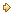Visitors Online: 77 | Sunday 20th October 2019

CBSE Guess > Papers > Question Papers > Class XII > 2004 > Economics > Outside Delhi Set-III

ECONOMICS (Set III—Outside Delhi)

Except for the following questions, all the remaining questions have been asked in Set I and Set II.

SECTION - A

Q. 1. Answer the following questions: 1x4
(i) Define marginal revenue.
(ii) In which market forni is there product differentiation?
(iii) State the law of supply.
(iv) Define market demand.

Q. 2. State any three main features of perfect competition. Describe any one. 3

Q. 9. Complete the following table: 4

 Output (unit) Marginal Revenue (Rs.) Total Revenue (Rs.) Average Revenue (Rs.) 1 2 3 4 10 8 0 -2 — — — — — — — —

SECTION - B

Q. 13. Answer the following questions: 1x4

1. Which two transactions determine balance of trade?
2. What is macro-economics?
3. Define primary deficit.
4. Give an example of a micro-economic study.

Q. 14. What is the basis of classifying taxes into direct tax and indirect tax? Give an example of each. 3

Q. 15. Calculate Net National Disposable Income from the following data: 3

 Rs. (Crores) (i) Gross domestic product at market price (ii) Net factor income from abroad (iii) Consumption of fixed capital (iv) Net current transfers from rest of the world (v) Net indirect taxes 1,500 (-) 20 100 (-) 30 120

Q. 16. In an economy, the marginal propensity to consume is 0.8. Investment is increased by Rs. 500 crores. Calculate the total increase in income and consumption expenditures. 3

Q. 24. From the following data calculate National Income by (i) income method and (ii) expenditure method: 3,3

 Rs. (Crores) (i) Private final consumption expenditure (ii) Net domestic capital formation (iii) Compensation of employees (iv) Mixed income of self-employed (v) Government final consumption expenditure (vi) Net factor income from abroad (vii) Profits (viii) Rent (ix) Net exports (x) Interest (xi) Net indirect tax (xii) Net current transfers from rest of the world 900 200 500 400 400 (-) 10 220 90 (-) 25 100 165 50

 Economics 2004 Question Papers Class XII Delhi Outside Delhi Compartment Delhi Compartment Outside DelhiSet ISet ISet ISet ISet IISet IISet IISet IISet IIICBSE 2004 Question Papers Class XII